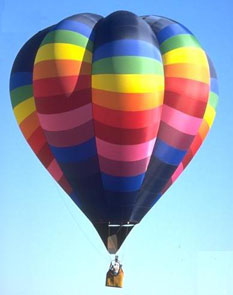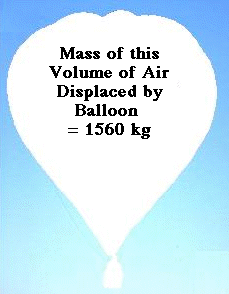+ Text Only Site
+ Non-Flash Version
+ Contact GlennF = 12,387 Newtons

Steps to complete the problem:
 List all of the variables given. Mass of balloon = 80 kg Volume of balloon = 1200 m3 Density of Helium = .18 kg/m3 Density of Air = 1.3 kg/m3 Determine the the total mass that can be lifted by the helium balloon. Mass lifted = mass of the volume of fluid displaced Mass = (1200 m3)( 1.3 kg/m3) Mass = 1560 kg The total amount that can be lifted = 1560 kg (including balloon, helium and payload)Determine the mass that is displaced. Determine the mass of helium. (1200 m3)*(.18 kg/m3) = 216 kg Subtract the mass of the balloon and mass of the 1200 m3 volume of helium from the total mass that can be lifted. 1560 kg - 80 kg - 216 kg = 1264 kg Determine the Force required to lift the displace volume of 1200 m3. F = mg (where g is gravity) F = (1264 kg) * (9.8 m/s2) F = 12,387 Newtons+ Inspector General Hotline + Equal Employment Opportunity Data Posted Pursuant to the No Fear Act + Budgets, Strategic Plans and Accountability Reports + Freedom of Information Act + The President's Management Agenda + NASA Privacy Statement, Disclaimer, and Accessibility CertificationEditor: Tom Benson NASA Official: Tom Benson Last Updated: May 13 2021 + Contact Glenn